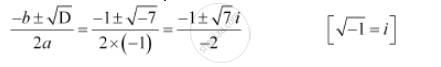CBSE (Arts) Class 11CBSE
Share

# Solve the Equation –X2 + X – 2 = 0 - CBSE (Arts) Class 11 - Mathematics

#### Question

Solve the equation –x2 + x – 2 = 0

#### Solution

The given quadratic equation is –x2 + – 2 = 0

On comparing the given equation with ax2 + bx + c = 0, we obtain

a = –1, b = 1, and c = –2

Therefore, the discriminant of the given equation is

D = b2 – 4ac = 12 – 4 × (–1) × (–2) = 1 – 8 = –7

Therefore, the required solutions areIs there an error in this question or solution?

#### Video TutorialsVIEW ALL 

Solution Solve the Equation –X2 + X – 2 = 0 Concept: Quadratic Equations.
S